# CPU Scheduling MCQ Quiz - 1

## 30 Questions MCQ Test RRB JE for Computer Science Engineering | CPU Scheduling MCQ Quiz - 1

Description
This mock test of CPU Scheduling MCQ Quiz - 1 for Computer Science Engineering (CSE) helps you for every Computer Science Engineering (CSE) entrance exam. This contains 30 Multiple Choice Questions for Computer Science Engineering (CSE) CPU Scheduling MCQ Quiz - 1 (mcq) to study with solutions a complete question bank. The solved questions answers in this CPU Scheduling MCQ Quiz - 1 quiz give you a good mix of easy questions and tough questions. Computer Science Engineering (CSE) students definitely take this CPU Scheduling MCQ Quiz - 1 exercise for a better result in the exam. You can find other CPU Scheduling MCQ Quiz - 1 extra questions, long questions & short questions for Computer Science Engineering (CSE) on EduRev as well by searching above.
QUESTION: 1

### Consider three processes (process id 0, 1, 2 respectively) with compute time bursts 2, 4 and 8 time units. All processes arrive at time zero. Consider the longest remaining time first (LRTF) scheduling algorithm. In LRTF ties are broken by giving priority to the process with the lowest process id. The average turn around time is:

Solution:

Let the processes be p0, p1 and p2. These processes will be executed in following order.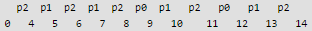Turn around time of a process is total time between submission of the process and its completion. Turn around time of p0 = 12 (12-0) Turn around time of p1 = 13 (13-0) Turn around time of p2 = 14 (14-0) Average turn around time is (12+13+14)/3 = 13.

QUESTION: 2

### Consider three processes, all arriving at time zero, with total execution time of 10, 20 and 30 units, respectively. Each process spends the first 20% of execution time doing I/O, the next 70% of time doing computation, and the last 10% of time doing I/O again. The operating system uses a shortest remaining compute time first scheduling algorithm and schedules a new process either when the running process gets blocked on I/O or when the running process finishes its compute burst. Assume that all I/O operations can be overlapped as much as possible. For what percentage of time does the CPU remain idle?

Solution:

Let three processes be p0, p1 and p2. Their execution time is 10, 20 and 30 respectively. p0 spends first 2 time units in I/O, 7 units of CPU time and finally 1 unit in I/O. p1 spends first 4 units in I/O, 14 units of CPU time and finally 2 units in I/O. p2 spends first 6 units in I/O, 21 units of CPU time and finally 3 units in I/O.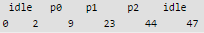Total time spent = 47 Idle time = 2 + 3 = 5 Percentage of idle time = (5/47)*100 = 10.6 %

QUESTION: 3

### Consider three CPU-intensive processes, which require 10, 20 and 30 time units and arrive at times 0, 2 and 6, respectively. How many context switches are needed if the operating system implements a shortest remaining time first scheduling algorithm? Do not count the context switches at time zero and at the end.

Solution:

Let three process be P0, P1 and P2 with arrival times 0, 2 and 6 respectively and CPU burst times 10, 20 and 30 respectively. At time 0, P0 is the only available process so it runs. At time 2, P1 arrives, but P0 has the shortest remaining time, so it continues. At time 6, P2 arrives, but P0 has the shortest remaining time, so it continues. At time 10, P1 is scheduled as it is the shortest remaining time process. At time 30, P2 is scheduled. Only two context switches are needed. P0 to P1 and P1 to P2.

QUESTION: 4

Which of the following process scheduling algorithm may lead to starvation

Solution:

Shortest job next may lead to process starvation for processes which will require a long time to complete if short processes are continually added.

QUESTION: 5

If the quantum time of round robin algorithm is very large, then it is equivalent to:

Solution:

If time quantum is very large, then scheduling happens according to FCFS.

QUESTION: 6

A scheduling algorithm assigns priority proportional to the waiting time of a process. Every process starts with priority zero (the lowest priority). The scheduler re-evaluates the process priorities every T time units and decides the next process to schedule. Which one of the following is TRUE if the processes have no I/O operations and all arrive at time zero?

Solution:

The scheduling algorithm works as round robin with quantum time equals to T. After a process's turn comes and it has executed for T units, its waiting time becomes least and its turn comes again after every other process has got the token for T units.

QUESTION: 7

Consider the 3 processes, P1, P2 and P3 shown in the table.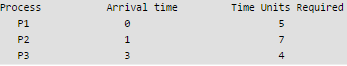Solution:

FCFS is clear. In RR, time slot is of 2 units. Processes are assigned in following order p1, p2, p1, p3, p2, p1, p3, p2, p2

This question involves the concept of ready queue. At t=2, p2 starts and p1 is sent to the ready queue and at t=3 p3 arrives so then the job p3 is queued in ready queue after p1. So at t=4, again p1 is executed then p3 is executed for first time at t=6.

QUESTION: 8

Consider the following table of arrival time and burst time for three processes P0, P1 and P2.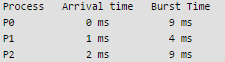The pre-emptive shortest job first scheduling algorithm is used. Scheduling is carried out only at arrival or completion of processes. What is the average waiting time for the three processes?

Solution:
QUESTION: 9

Which of the following statements are true?

I. Shortest remaining time first scheduling may cause starvation
II. Preemptive scheduling may cause starvation
III. Round robin is better than FCFS in terms of response time

Solution:

I) Shortest remaining time first scheduling is a pre-emptive version of shortest job scheduling. In SRTF, job with the shortest CPU burst will be scheduled first. Because of this process, It may cause starvation as shorter processes may keep coming and a long CPU burst process never gets CPU.
II) Pre-emptive just means a process before completing its execution is stopped and other process can start execution. The stopped process can later come back and continue from where it was stopped. In pre-emptive scheduling, suppose process P1 is executing in CPU and after some time process P2 with high priority then P1 will arrive in ready queue then p1 is pre-empted and p2 will brought into CPU for execution. In this way if process which is arriving in ready queue is of higher priority then p1, then p1 is always pre-empted and it may possible that it suffer from starvation.
III) round robin will give better response time then FCFS ,in FCFS when process is executing ,it executed up to its complete burst time, but in round robin it will execute up to time quantum. So Round Robin Scheduling improves response time as all processes get CPU after a specified time. So, I,II,III are true which is option (D). Option (D) is correct answer.

QUESTION: 10

In the following process state transition diagram for a uniprocessor system, assume that there are always some processes in the ready state: Now consider the following statements: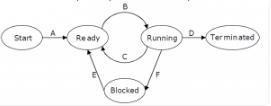I. If a process makes a transition D, it would result in another process making transition A immediately.

II. A process P2 in blocked state can make transition E while another process P1 is in running state.

III. The OS uses preemptive scheduling.

IV. The OS uses non-preemptive scheduling.

Q. Which of the above statements are TRUE?

Solution:

I is false. If a process makes a transition D, it would result in another process making transition B, not A. II is true. A process can move to ready state when I/O completes irrespective of other process being in running state or not. III is true because there is a transition from running to ready state. IV is false as the OS uses preemptive scheduling.

QUESTION: 11

Group 1 contains some CPU scheduling algorithms and Group 2 contains some applications. Match entries in Group 1 to entries in Group 2.

Group I                                                                    Group II
(P) Gang Scheduling                                      (1) Guaranteed Scheduling
(Q) Rate Monotonic Scheduling                     (2) Real-time Scheduling
(R) Fair Share Scheduling                              (3) Thread Scheduling

Solution:
QUESTION: 12

An operating system uses Shortest Remaining Time first (SRT) process scheduling algorithm. Consider the arrival times and execution times for the following processes:

Process                   Execution time                      Arrival time
P1                              20                                              0
P2                              25                                              15
P3                              10                                              30
P4                              15                                              45

Q. What is the total waiting time for process P2?

Solution:

Shortest remaining time, also known as shortest remaining time first (SRTF), is a scheduling method that is a pre-emptive version of shortest job next scheduling. In this scheduling algorithm, the process with the smallest amount of time remaining until completion is selected to execute. Since the currently executing process is the one with the shortest amount of time remaining by definition, and since that time should only reduce as execution progresses, processes will always run until they complete or a new process is added that requires a smaller amount of time. The Gantt chart of execution of processes: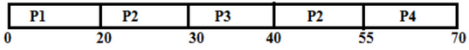At time 0, P1 is the only process, P1 runs for 15 time units. At time 15, P2 arrives, but P1 has the shortest remaining time. So P1 continues for 5 more time units. At time 20, P2 is the only process. So it runs for 10 time units. at time 30, P3 is the shortest remaining time process. So it runs for 10 time units. at time 40, P2 runs as it is the only process. P2 runs for 5 time units. At time 45, P3 arrives, but P2 has the shortest remaining time. So P2 continues for 10 more time units. P2 completes its execution at time 55.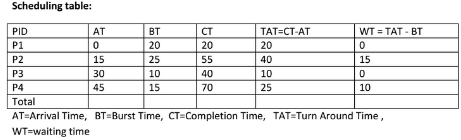As we know, turn around time is total time between submission of the process and its completion. Waiting time is the time The amount of time that is taken by a process in ready queue and waiting time is the difference between Turn around time and burst time. Total turnaround time for P2 = Completion time - Arrival time = 55 - 15 = 40 Total Waiting Time for P2= turn around time - Burst time = 40 – 25 = 15

QUESTION: 13

Consider three CPU-intensive processes, which require 10, 20 and 30 time units and arrive at times 0, 2 and 6, respectively. How many context switches are needed if the operating system implements a shortest remaining time first scheduling algorithm? Do not count the context switches at time zero and at the end.

Solution:

Shortest remaining time, also known as shortest remaining time first (SRTF), is a scheduling method that is a pre-emptive version of shortest job next scheduling. In this scheduling algorithm, the process with the smallest amount of time remaining until completion is selected to execute. Since the currently executing process is the one with the shortest amount of time remaining by definition, and since that time should only reduce as execution progresses, processes will always run until they complete or a new process is added that requires a smaller amount of time. Solution: Let three process be P0, P1 and P2 with arrival times 0, 2 and 6 respectively and CPU burst times 10, 20 and 30 respectively. At time 0, P0 is the only available process so it runs. At time 2, P1 arrives, but P0 has the shortest remaining time, so it continues. At time 6, P2 also arrives, but P0 still has the shortest remaining time, so it continues. At time 10, P1 is scheduled as it is the shortest remaining time process. At time 30, P2 is scheduled. Only two context switches are needed. P0 to P1 and P1 to P2.

QUESTION: 14

Three processes A, B and C each execute a loop of 100 iterations. In each iteration of the loop, a process performs a single computation that requires tc CPU milliseconds and then initiates a single I/O operation that lasts for tio milliseconds. It is assumed that the computer where the processes execute has sufficient number of I/O devices and the OS of the computer assigns different I/O devices to each process. Also, the scheduling overhead of the OS is negligible. The processes have the following characteristics:

Process id                                         tc                        tio
A                                                       100 ms                500 ms
B                                                        350 ms               500 ms
C                                                        200 ms               500 ms

Q. The processes A, B, and C are started at times 0, 5 and 10 milliseconds respectively, in a pure time sharing system (round robin scheduling) that uses a time slice of 50 milliseconds. The time in milliseconds at which process C would complete its first I/O operation is ___________.

Solution:

There are three processes A, B and C that run in round robin manner with time slice of 50 ms.
Processes atart at 0, 5 and 10 miliseconds.

The processes are executed in below order
A, B, C, A
50 + 50 + 50 + 50 (200 ms passed)

Now A has completed 100 ms of computations and goes for I/O now
B, C, B, C, B, C
50 + 50 + 50 + 50 + 50 + 50 (300 ms passed)

C goes for i/o at 500ms and it needs 500ms to finish the IO.

So C would complete its first IO at 1000 ms

QUESTION: 15

An operating system uses shortest remaining time first scheduling algorithm for pre-emptive scheduling of processes. Consider the following set of processes with their arrival times and CPU burst times (in milliseconds):

Process Arrival                           Time Burst          Time
P1                                                   0                         12
P2                                                   2                          4
P3                                                   3                          6
P4                                                   8                          5

Q. The average waiting time (in milliseconds) of the processes is _________.

Solution:

Process         Arrival Time     Burst Time
P1                           0                      12
P2                            2                       4
P3                            3                       6
P4                            8                       5

Burst Time - The total time needed by a process from the CPU for its complete execution. Waiting Time - How much time processes spend in the ready queue waiting their turn to get on the CPU Now, The Gantt chart for the above processes is :

P1 - 0 to 2 milliseconds
P2 - 2 to 6 milliseconds
P3 - 6 to 12 milliseconds
P4 - 12 to 17 milliseconds
P1 - 17 to 27 milliseconds

Process p1 arrived at time 0, hence cpu started executing it. After 2 units of time P2 arrives and burst time of P2 was 4 units, and the remaining time of the process p1 was 10 units,hence cpu started executing P2, putting P1 in waiting state(Pre-emptive and Shortest remaining time first scheduling). Due to P1's highest remaining time it was executed by the cpu in the end.

Now calculating the waiting time of each process:
P1 -> 17 -2 = 15
P2 -> 0
P3 -> 6 - 3 = 3
P4 -> 12 - 8 = 4

Hence total waiting time of all the processes is = 15+0+3+4=22
Total no of processes = 4
Average waiting time = 22 / 4 = 5.5

QUESTION: 16

Consider the following set of processes, with the arrival times and the CPU-burst times given in milliseconds

Process                          Arrival Time                    Burst Time
P1                                           0                                        5
P2                                           1                                        3
P3                                           2                                        3
P4                                           4                                        1

Q.What is the average turnaround time for these processes with the preemptive shortest remaining processing time first (SRPT) algorithm ?

Solution:

The following is Gantt Chart of execution

P1           P2            P4             P3        P1
1               4               5               8         12

Turn Around Time = Completion Time - Arrival Time   Avg Turn Around Time  =  (12 + 3 + 6+  1)/4 = 5.50

QUESTION: 17

A uni-processor computer system only has two processes, both of which alternate 10ms CPU bursts with 90ms I/O bursts. Both the processes were created at nearly the same time. The I/O of both processes can proceed in parallel. Which of the following scheduling strategies will result in the least CPU utilization (over a long period of time) for this system ?

Solution:

When Round Robin scheduling is used We are given that the time slice is 5ms. Consider process P and Q. Say P utilizes 5ms of CPU and then Q utilizes 5ms of CPU. Hence after 15ms P starts with I/O And after 20ms Q also starts with I/O. Since I/O can be done in parallel, P finishes IO at 105th ms (15 + 90) and Q finishes its IO at 110th ms (20 + 90). Therefore we can see that CPU remains idle from 20th to 105th ms. That is when Round Robin scheduling is used, Idle time of CPU = 85ms CPU Utilization = 20/105 = 19.05% When First Come First Served scheduling scheduling or Shortest Remaining Time First is used Say P utilizes 10ms of CPU and then starts its I/O. At 11th ms Q starts processing. Q utilizes 10ms of CPU. P completes its I/O at 100ms (10 + 90) Q completes its I/O at 110ms (20 + 90) At 101th ms P again utilizes CPU. Hence, Idle time of CPU = 80ms CPU Utilization = 20/100 = 20% Since only two processes are involved and IO time is much more than CPU time, "Static priority scheduling with different priorities" for the two processes reduces to FCFS or Shortest remaining time first. Therefore, Round robin will result in least CPU utilization.

QUESTION: 18

Which of the following scheduling algorithms is non-preemptive?

Solution:

Round Robin - Preemption takes place when the time quantum expires First In First Out - No Preemption, the process once started completes before the other process takes over Multi Level Queue Scheduling - Preemption takes place when a process of higher priority arrives Multi Level Queue Scheduling with Feedback - Preemption takes a place when process of higher priority arrives or when the quantum of high priority queue expires and we need to move the process to low priority queue So, B is the correct choice.   Please comment below if you find anything wrong in the above post.

QUESTION: 19

Consider a set of n tasks with known runtimes r1, r2, .... rn to be run on a uniprocessor machine. Which of the following processor scheduling algorithms will result in the maximum throughput?

Solution:

Throughput means total number of tasks executed per unit time i.e. sum of waiting time and burst time.
Shortest job first scheduling is a scheduling policy that selects the waiting process with the smallest execution time to execute next.
Thus, in shortest job first scheduling, shortest jobs are executed first. This means CPU utilization is maximum. So, maximum number of tasks are completed.

Thus, option (B) is correct.

Please comment below if you find anything wrong in the above post.

QUESTION: 20

Consider a uniprocessor system executing three tasks T1, T2 and T3, each of which is composed of an infinite sequence of jobs (or instances) which arrive periodically at intervals of 3, 7 and 20 milliseconds, respectively. The priority of each task is the inverse of its period and the available tasks are scheduled in order of priority, with the highest priority task scheduled first. Each instance of T1, T2 and T3 requires an execution time of 1, 2 and 4 milliseconds, respectively. Given that all tasks initially arrive at the beginning of the 1st milliseconds and task preemptions are allowed, the first instance of T3 completes its execution at the end of ______________ milliseconds.

Solution:

Periods of T1, T2 and T3 are 3ms, 7ms and 20ms
Since priority is inverse of period, T1 is the highest priority task, then T2 and finally T3
Every instance of T1 requires 1ms, that of T2 requires 2ms and that of T3 requires 4ms
Initially all T1, T2 and T3 are ready to get processor, T1 is preferred
Second instances of T1, T2, and T3 shall arrive at 3, 7, and 20 respectively.
Third instance of T1, T2 and T3 shall arrive at 6, 14, and 49 respectively.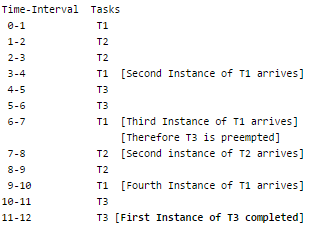QUESTION: 21

The maximum number of processes that can be in Ready state for a computer system with n CPUs is

Solution:

The size of ready queue doesn't depend on number of processes. A single processor system may have a large number of processes waiting in ready queue.

QUESTION: 22

For the processes listed in the following table, which of the following scheduling schemes will give the lowest average turnaround time?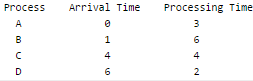Solution:

Turnaround time is the total time taken between the submission of a program/process/thread/task (Linux) for execution and the return of the complete output to the customer/user. Turnaround Time = Completion Time - Arrival Time. FCFS = First Come First Serve (A, B, C, D) SJF = Non-preemptive Shortest Job First (A, B, D, C) SRT = Shortest Remaining Time (A(3), B(1), C(4), D(2), B(5)) RR = Round Robin with Quantum value 2 (A(2), B(2), A(1),C(2),B(2),D(2),C(2),B(2)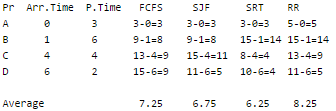Shortest Remaining Time produces minimum average turn-around time.

QUESTION: 23

Which of the following is FALSE about SJF (Shortest Job First Scheduling)?

S1: It causes minimum average waiting time
S2: It can cause starvation

Solution:
1. Both SJF and Shortest Remaining time first algorithms may cause starvation. Consider a situation when long process is there in ready queue and shorter processes keep coming.
2. SJF is optimal in terms of average waiting time for a given set of processes, but problems with SJF is how to know/predict time of next job.
QUESTION: 24

Two concurrent processes P1 and P2 use four shared resources R1, R2, R3 and R4, as shown below.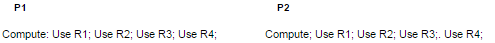Both processes are started at the same time, and each resource can be accessed by only one process at a time The following scheduling constraints exist between the access of resources by the processes:

• P2 must complete use of R1 before P1 gets access to R1
• P1 must complete use of R2 before P2 gets access to R2.
• P2 must complete use of R3 before P1 gets access to R3.
• P1 must complete use of R4 before P2 gets access to R4.

There are no other scheduling constraints between the processes. If only binary semaphores are used to enforce the above scheduling constraints, what is the minimum number of binary semaphores needed?

Solution:

P1:

Compute;
Wait(A);
Use R1;
Use R2;
Signal(B);
Wait(A);
Use R3;
Use R4;
Signal(B);

P2:

Compute;
Wait(B);
Use r1;
Signal(A);
Wait(B);
Use R2;
Use R3;
Signal(A);
Wait(B);
Use R4;
Signal(B);

In process p1, initially control will be stuck in while loop of Wait(A) because A = 0. In process p2, Wait(B) decrements the value of B to 0 . Now, P2 uses the resource R1 and increments the value to A to 1 so that process P1 can enter its critical section and use resource R1.
Thus, P2 will complete use of R1 before P1 gets access to R1.
Now, in P2 values of B = 0. So, P2 can not use resource R2 till P1 uses R2 and calls function Signal(B) to increment the value of B to 1. Thus, P1 will complete use of R2 before P2 gets access to R2.
Now, semaphore A = 0. So, P1 can not execute further and gets stuck in while loop of function Wait(A). Process P2 uses R3 and increments the value of semaphore A to 1.Now, P1 can enter its critical section to use R3. Thus, P2 will complete use of R3 before P1 gets access to R3.
Now, P1 will use R4 and increments the value of B to 1 so that P2 can enter is critical section to use R4. Thus, P1 will complete use of R4 before P2 gets access to R4.
Thus, option (B) is correct.
Please comment below if you find anything wrong in the above post.

QUESTION: 25

We wish to schedule three processes P1, P2 and P3 on a uniprocessor system. The priorities, CPU time requirements and arrival times of the processes are as shown below.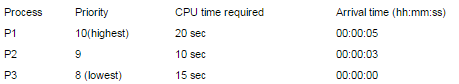We have a choice of preemptive or non-preemptive scheduling. In preemptive scheduling, a late-arriving higher priority process can preempt a currently running process with lower priority. In non-preemptive scheduling, a late-arriving higher priority process must wait for the currently executing process to complete before it can be scheduled on the processor. What are the turnaround times (time from arrival till completion) of P2 using preemptive and non-preemptive scheduling respectively.

Solution: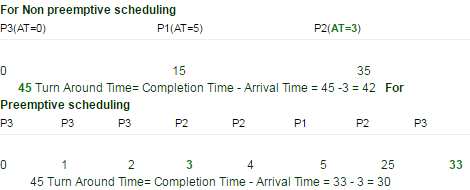QUESTION: 26

Consider an arbitrary set of CPU-bound processes with unequal CPU burst lengths submitted at the same time to a computer system. Which one of the following process scheduling algorithms would minimize the average waiting time in the ready queue?

Solution:

Turnaround time is the total time taken by the process between starting and the completion and waiting time is the time for which process is ready to run but not executed by CPU scheduler. As we know, in all CPU Scheduling algorithms, shortest job first is optimal i.ie. it gives minimum turn round time, minimum average waiting time and high throughput and the most important thing is that shortest remaining time first is the pre-emptive version of shortest job first. shortest remaining time first scheduling algorithm may lead to starvation because If the short processes are added to the cpu scheduler continuously then the currently running process will never be able to execute as they will get pre-empted but here all the processes are arrived at same time so there will be no issue such as starvation. So, the answer is Shortest remaining time first, which is answer (A).

QUESTION: 27

Consider the following processes, with the arrival time and the length of the CPU burst given in milliseconds. The scheduling algorithm used is preemptive shortest remaining-time first.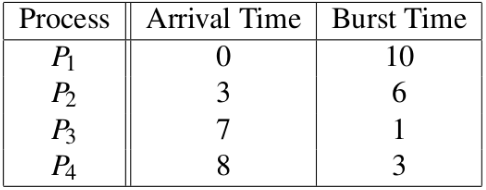The average turn around time of these processes is ___________ milliseconds.   Note : This question was asked as Numerical Answer Type.

Solution:

PreEmptive Shortest Remaining time first scheduling, i.e. that processes will be scheduled on the CPU which will be having least remaining burst time( required time at the CPU). The processes are scheduled and executed as given in the below Gantt chart.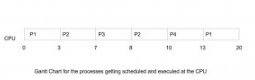Turn Around Time(TAT) = Completion Time(CT) - Arrival Time(AT) TAT for P1 = 20 - 0 = 20 TAT for P2 = 10 - 3 = 7 TAT for P3 = 8- 7 = 1 TAT for P4 = 13 - 8 = 5 Hence, Average TAT = Total TAT of all the processes / no of processes = ( 20 + 7 + 1 + 5 ) / 4 = 33 / 4 = 8.25   Thus, A is the correct choice.

QUESTION: 28

Consider n jobs J1, J2,......Jn such that job Ji has execution time ti and a non-negative integer weight wi. The weighted mean completion time of the jobs is defined to be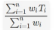, where Ti is the completion time of job Ji. Assuming that there is only one processor available, in what order must the jobs be executed in order to minimize the weighted mean completion time of the jobs?

Solution:
QUESTION: 29

Assume every process requires 3 seconds of service time in a system with single processor. If new processes are arriving at the rate of 10 processes per minute, then estimate the fraction of time CPU is busy in system?

Solution:

10 processes -> 1 min
1 process-> 1/10 min = 6 sec (Arrival rate)
Each process -> 3 sec service time 3/6 * 100 = 50% of time CPU is busy.

QUESTION: 30

The strategy of making processes that are logically runnable to be temporarily suspended is called :

Solution: